# ICSE Class 10 Maths Exam 2018: Question Paper Analysis

ICSE or Indian Council of Secondary Education is a private educational board which is known for its quality of education and standard exam pattern. ICSE conducted the 2018 Class 10 mathematics examination on Tuesday, 26th February. The exam duration was two and half hours and started at 11:00 a.m. According to most students, the mathematics question paper was of medium level difficult.

Check ICSE class 10 post paper analysis 2019 here.

### Question Pattern

The question pattern of the ICSE Class 10 maths was according to the guidelines of the Board. The mathematics exam paper had a time duration of 2 and a half hours with a total mark of 80. The examination started at sharp 11:00 a.m. on 26th February 2018. The maths question paper was divided into 2 sections namely Section A and Section B. The Section A consisted a total of 40 marks and Section B consisted a total of 40 marks. All questions were compulsory in Section A, whereas, there were options in Section B. The detailed question pattern of ICSE Class 10 mathematics exam is given in the table mentioned below.

 Section Attempt Marks distribution A All Compulsory 40 B Any four 40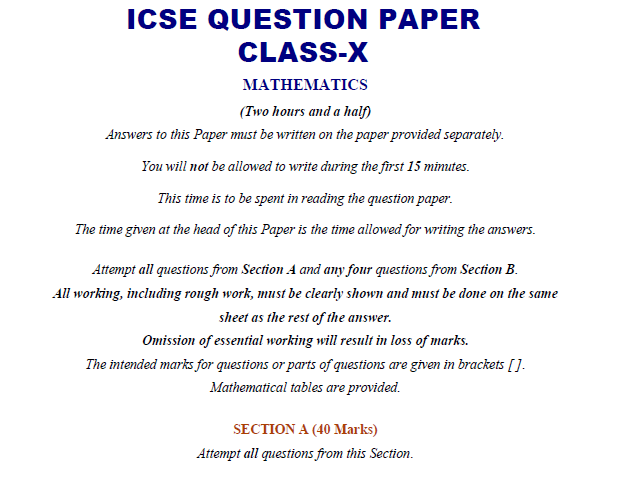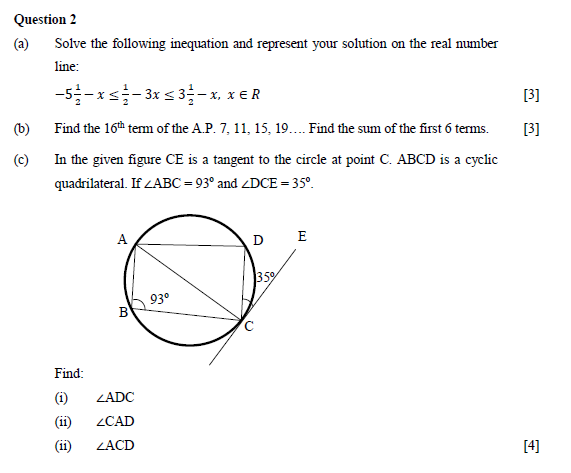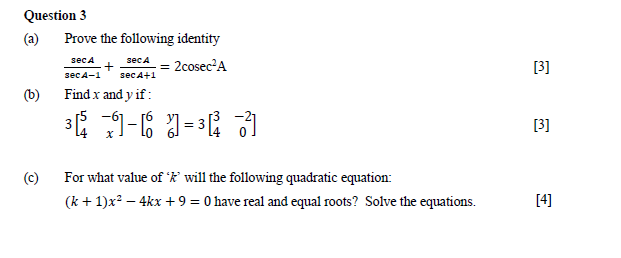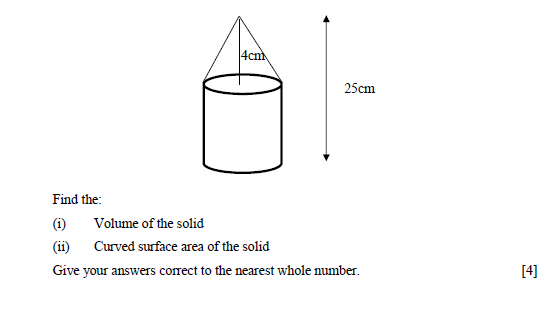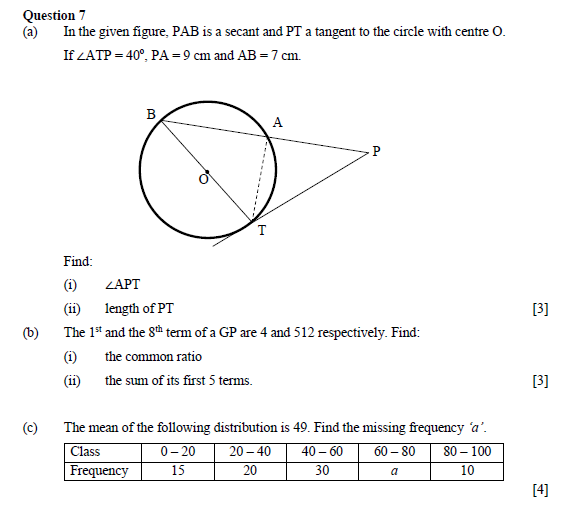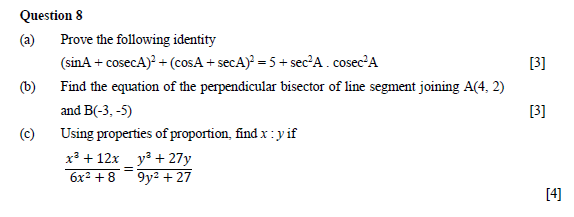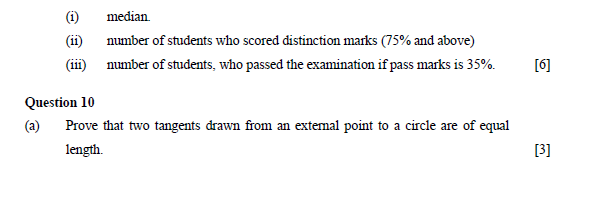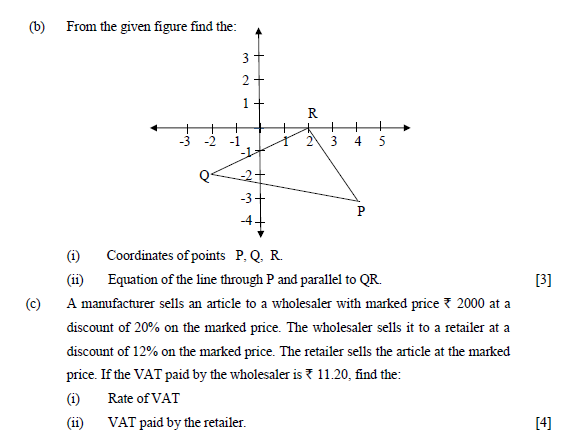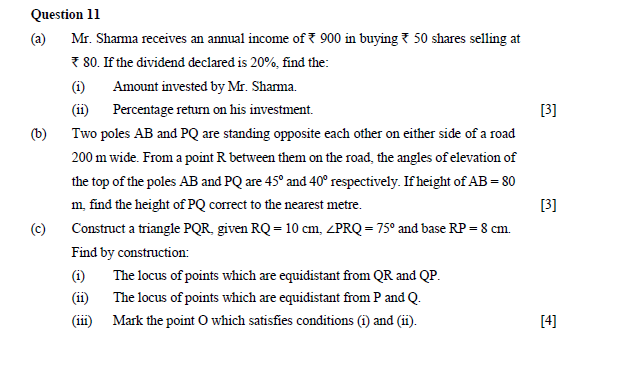### Chapter-Wise Marks Weightage

This year questions came from all sections in the mathematics examination.

• The Unit Algebra section had most questions asked from Quadratic Equations, Matrices, GP, AP, Factor Theorem, Remainder Theorem, and Linear Inequations.
• The Trigonometry section included problems from Identities and Applications.
• The section Statistics consisted questions from Median, Mean, Lower Quartile and Histogram.
• The equation of Lines, Section Formula, and Reflection came from the section Coordinate Geometry.
• Commercial Mathematics consisted problems from the topics Recurring Deposits and Shares and Dividends.
• The Mensuration section included problems on Cylinders, Combination of Solids, etc.

The chapter wise marks weightage, along with topic-wise weightage and the weightage percentage is given in the table mentioned below.

 Topics No. of Questions Weightage Percentage Marks Weightage Commercial Mathematics 3 8% 9 Coordinate Geometry 3 10% 11 Algebra 12 35% 38 Mensuration 2 6% 7 Geometry 5 16% 17 Statistics 4 15% 17 Trigonometry 3 10% 11

### ICSE Class 1O Maths Question Paper

#### Overview of ICSE Class 10 Maths Exam 2018

 Difficulty Level Easy Average Expected Score 90+ Question Weightage From all major topics Maximum Questions Algebra

### General Review

According to most students, the exam was easy with few questions of medium level. Students who have prepared well for the maths exam and practiced the ICSE sample papers on a regular basis can easily score above 90.

Stay tuned with BYJU’S to get the latest news and notification on ICSE Exam along with the exam pattern, the exam syllabus, marking scheme and other important details.

Check ICSE class 10 Physics 2019 post paper analysis here.